Classical Treatment of Doubly-Excited Helium Atoms

Introduction

Quantum mechanical analysis of the hydrogen atom is straightforward; it can be found in introductory quantum mechanics textbooks. However, when we increase the level of complexity by adding one more electron into the system, the problem suddenly becomes non-trivial. Helium atoms, consisting of a positively charged nucleus and two negatively charged electrons, have not allowed an exact solution. So far, only approximation techniques have been developed to predict the energy spectrum of helium atoms.

Doubly-excited helium atoms, the main focus of this report, do not even allow most of these well-established approximation techniques. This is because they are not in a bound state; both of the electrons in a doubly-excited helium atom are at energy levels higher than their ground state energies, which brings up the total energy in the system higher than the ionized states, He+ + e-. Therefore, this state is unstable and will eventually ionize after a certain period of time.

Despite the fact that the doubly-excited state of helium atoms is only transient, the theoretical description of this state and its dynamics is an important key to understanding the scattering properties of helium atoms. Many novel approaches describing the doubly-excited state of helium atoms have been discussed from the quantum viewpoint (e.g. see [1,2]), while at the same time, semi-classical and purely classical approaches have made great progress in this area of research as well . In this report, I will concentrate on the analysis of a particular state of helium, commonly known as the Z2+e-e- configuration, using classical mechanics.

Coulomb Three-Body Problem

The helium atom is a three-body coulomb system. Electrons are held in orbit by the attractive coulomb force of a massive nucleus. It resembles the famous three-body problem in astrophysics such as the Sun-Earth-Moon system. However, a system of a helium atom is different from the typical three-body problem in that

1. the two electrons exert repulsive forces on each other
2. the two orbiting electrons have comparable (in fact, equal) masses

Classical analysis of helium atoms is called the Coulomb three-body problem, and as in the gravitational three-body problem, Coulomb three-body problem has no analytic solution. However, the problem can be simplified using a couple of approximation schemes before it is solved numerically.

In the most general case, the dynamics of three bodies can be described by an 18-dimensional phase-space, and the Hamiltonian for the system is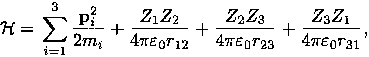where Zi is the charge of the i-th particle.

Using the fact that the nucleus is much heavier than the electron, we can approximate the nucleus mass as infinite and define the position of the nucleus (which is now the center of the mass) to be at the origin of the coordinate system. This reduces the problem to six degrees of freedom (12 dimensions in phase space). Using atomic units (e = me = 1) and a proper length scale, the Hamiltonian becomes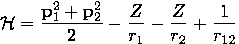Finally, we focus on the special case of a system with zero angular momentum (L= 0). There have been many studies on zero angular momentum three-body systems simply because this reduces the complexity of the problem a great deal. In the study of highly-excited helium atoms such a choice is not entirely arbitrary, but rather, it is actually a good approximation. For the Hamiltonian above, we are free to apply a scaling transformation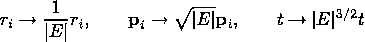which normalizes the problem to an energy-independent form,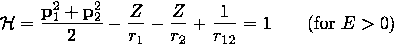But this transformation also rescales the angular momentum L as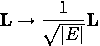Therefore, highly-excited helium atoms can be approximated by L= 0. Now, as proved in , three-body systems with zero angular momentum must have a planetary configuration. That is, the movements of its constituents are confined in a two dimensional plane. This cuts down the degree of freedom of our problem to three, namely three scalar variables r1, r2 and r12.

Zee Configuration

Now that we have reduced the complexity of the problem to three degrees of freedom, it is not very difficult to simulate all types of trajectories of electrons inside a helium atom using a computer. However, instead of considering all possible (classical) motions of the electrons inside the doubly-excited helium atoms, we focus here on a certain group of motions that are more interesting, namely, what is called the Z2+e-e- configuration.

The Hamiltonian above reveals that there are two discrete symmetries, one associated with the reflection, (r1, r2) &rarr (-r1, -r2), and the other associated to the particle exchange transformation, (r1, r2) &rarr (r2,r1). This gives rise to an invariant subspaces inside the six dimensional phase space in which trajectories that start in one of those subspaces remain there for all times. Examples of such subspaces are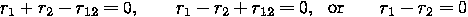Trajectories that lie on r1 + r2 - r12 = 0 and r1 - r2 + r12 = 0 subspace describe helium atoms in which two electrons are orbiting around the nucleus forming a collinear arrangement. r1 + r2 - r12= 0 is the state where two electrons are on opposite sides of the nucleus (eZe), and r1 - r2 + r12= 0 is where two electrons are on the same side of the nucleus (Zee). The third example, r1 - r2= 0, describes trajectories where the two electrons stay equidistance from the nucleus at all times (Wannier ridge ).

Of the three simplest examples of symmetric motions given above, only the "Zee" case, r1 - r2 + r12= 0, is a stable trajectory for the electrons. Simulations show that other symmetric motions are chaotic in nature and soon lead to autoionization .

The Zee state has many interesting features, other than the fact that two electrons are orbiting the neutron with the same frequency (collinear arrangement). First of all, the two electrons are always distinguishable as the inner electron and the outer electron. These two cannot swap their positions because their degrees of freedom are confined to one dimension and they remain separated due to their repulsive forces on each other.

Depending on the initial conditions, the inner electron exhibits varying degrese of elliptical motions, but the distance between the outer electron and the nucleus converges to a stable value over time. Since the outer electron stays nearly fixed when viewed in a rotational frame, it is usually referred to as a "frozen planet orbit."

Another critical feature the Zee state has is its robustness against perturbations. Not only does it maintain stability when the initial conditions are varied within the symmetry constraints, r1 - r2 + r12= 0, it is also stable when it is perturbed away from the above subspace, or even when the initial assumption L = 0 is slightly relaxed. This is a strong indication that this Zee state occurs in Nature.

Application of the Classical Idea

The notion of the frozen planet orbit has given birth to a new approximation technique within quantum mechanics that explains the energy levels of two-electron atoms. This technique utilizes the fact that the outer electron is almost fixed in space, thus only weakly coupled with other electron and the nucleus. The state of this frozen electron is approximated as an harmonic-oscillator and therefore it can be quantized via normal modes. Parameters used in this approximation are borrowed from the classical properties of the frozen planet orbit. It has been shown that this approximation is superior to other known approximations in a semi-classical limit (high energy) .

The idea of frozen planet orbit can be also found in spectroscopy on other helium-like atoms, which contain two electrons in their outermost shells such as Calcium (atomic number 20) and Barium (56). For example, in a laser spectroscopic study on Barium by Eichmann et al., Barium atoms were highly excited using six-laser beams into planetary states and after the autoionization their energies were measured. Eichmann notes that the resulting spectrum could be understood only when we assume that there is a "frozen electron."

© 2007 Yeong Dae Kwon. The author grants permission to copy, distribute and display this work in unaltered form, with attribution to the author, for noncommercial purposes only. All other rights, including commercial rights, are reserved to the author.

References

 C.E. Wulfman, "R4 Invariants for Doubly Excited States of Helium," Phys. Lett. A 26, 9 (1968).

 J. Macek, "Properties of Autoionizing States of He," J. Phys. B 1, 2 (1968).

 G. Tanner, "The Theory of Two-Electron Atoms: Between Ground State and Complete Fragmentation," Rev. Mod. Phys. 72 (2000).

 L.A. Pars, A Treaties on Analytical Dynamics (Heinemann, 1965).

 G.H. Wannier, "The Threshold Law for Single Ionization of Atoms or Ions by Electrons," Phys. Rev. 90, 5 (1953).

 D. Wintgen, K. Richter and G. Tanner, "Classical Mechanics of Two-Electron Atoms," Phys. Rev. A 48, 6 (1993).

 D. Wintgen, E.A. Soloven, K. Richter and J.S. Briggs, "Novel Dynamical Symmetries of Asymmetrically Doubly Excited Two-Electron Atoms," J. Phys. B 25 (1992).

 V. Lange, U. Eichmann and W. Sandner, "Positional Correlation in Laser-Excited Three-Body Coulomb Systems," Phys. Rev. Lett. 64, 3 (1990).